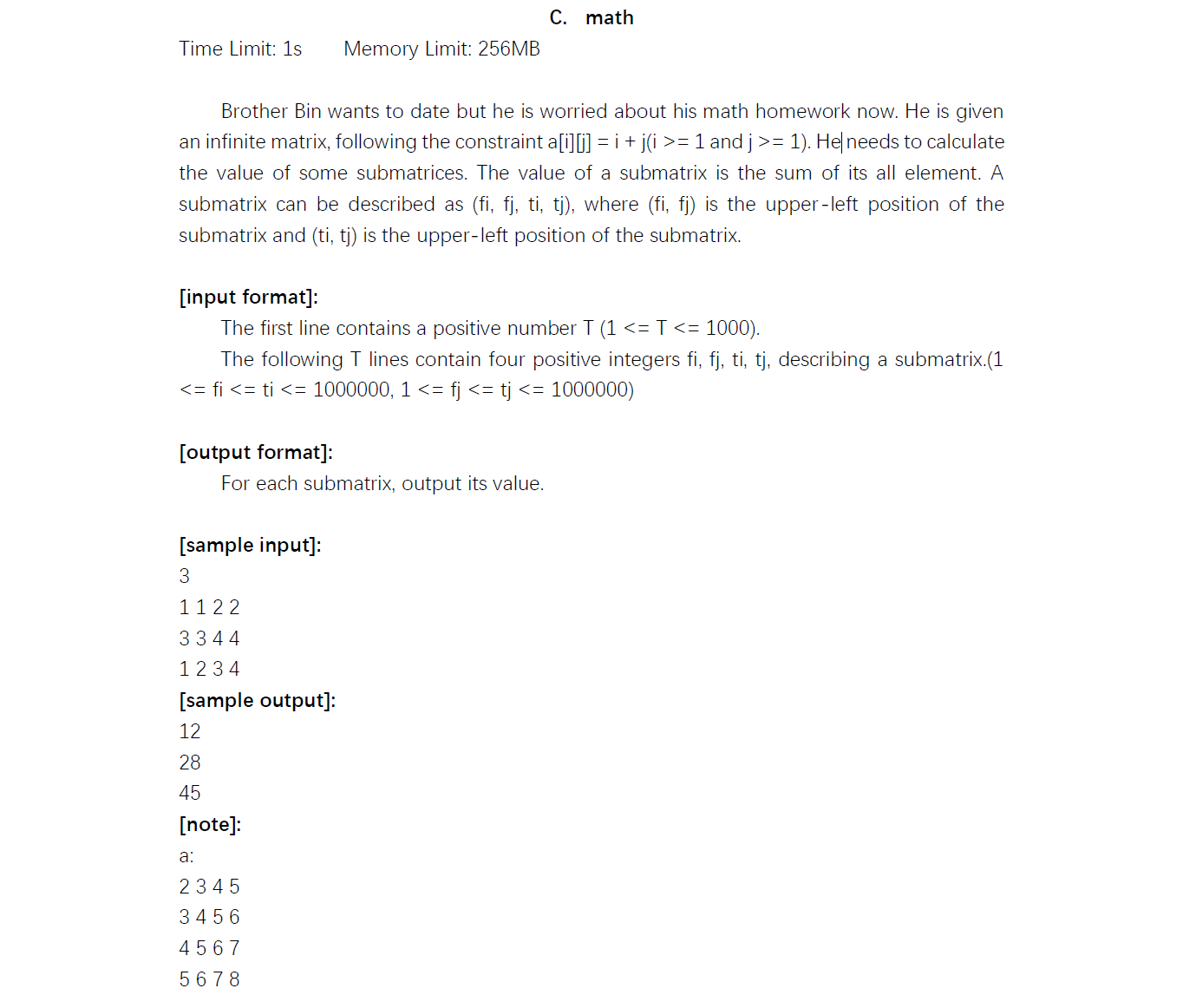# Halo

A magic place for coding

0%

## 题目

ACM网络赛的C题，考点是矩阵，题目如下：## 分析

题目本身比较容易理解，就是计算一个子矩阵的元素和。这个矩阵的每个元素的值是横纵坐标的和。

最基本的做法就是一个一个算，当然这种做法时间复杂度过高，肯定是做不了的。所以我们需要寻找另外的方法。

这个矩阵本身的构造给了我们很大的帮助，因为它的值的分布是有规律的，对于一个子矩阵来说，水平方向是公差为1的等差数列，竖直方向也是公差为1的等差数列。给定子矩阵的左上角坐标和右下角坐标，我们就能够知道这个等差数列的首项和末项，对于每一行应用等差数列求和公式，这个复杂度就降低了一点。

但是比赛的时候想着能不能直接做到$O(1)$的复杂度呢？经过分析，因为竖直方向上也是等差数列，我们将每一行的和看成是一个个体，实际上每一行的和也是一个等差数列，不过它们的公差是子矩阵的宽度。这样我们就可以依靠第一行的和，来计算下面每一行的值了。实际上，我们只需要计算下面每一行因为公差而增加的数，其余部分只需要用第一行的值乘上列数即可。

简单推导一下这个等差数列，假设子矩阵的宽度为$d$（即公比），那么第一行的值为0（以第一行为基准），第二行的值为$d$，第三行为$2d$，以此类推，第$n$行的值为$(n-1)d$，此时
$$S_n = (0 + (n-1)d) \times n / 2 \ = (n-1) \times n \times d / 2$$
按照题目给的假设，子矩阵左上角为$(f_i, f_j)$，右下角为$(t_i, t_j)$，我们推出下面的计算公式：
$$sum = 第一行的和=((f_i+f_j) + (f_i+t_j)) \times (t_j - f_j + 1) / 2$$
其中$f_i+f_j$是首项，$f_i+t_j$是末项，$(t_j - f_j + 1)$是列数，即矩阵的宽度。
$$sum += 第一行的和 \times (t_i - f_i) + (t_i - f_i + 1) \times (t_j - f_j + 1) \times (t_i - f_i) / 2$$
其中，$t_i-f_i$是行数-1（除去第一行已经计算的），$t_i-f_i+1$是公差（以每一行的和为元素的等差数列），$t_j - f_j + 1$是行数。

按照上述公式编程就能够得到正确答案，编程方面没什么难度。但是依然pass不了，于是我考虑到了是越界的问题，尝试了[1, 1, 1, 100000]这个输入，发现结果果然错了，于是修改数据类型，使用unsigned long long发现还是不行。因为计算的代码不多，所以我首先考虑的就是乘法的溢出问题，使用通用的大数乘法后，就能成功通过了。

## 小结

在处理矩阵的算法题时，要充分利用矩阵本身的性质来减少时间复杂度。矩阵的处理一般都是比较耗时的，我们可以通过用空间换取时间、利用矩阵数据特性的方法来降低时间复杂度。希望这篇博客能够帮助到你，谢谢！

Welcome to my other publishing channels